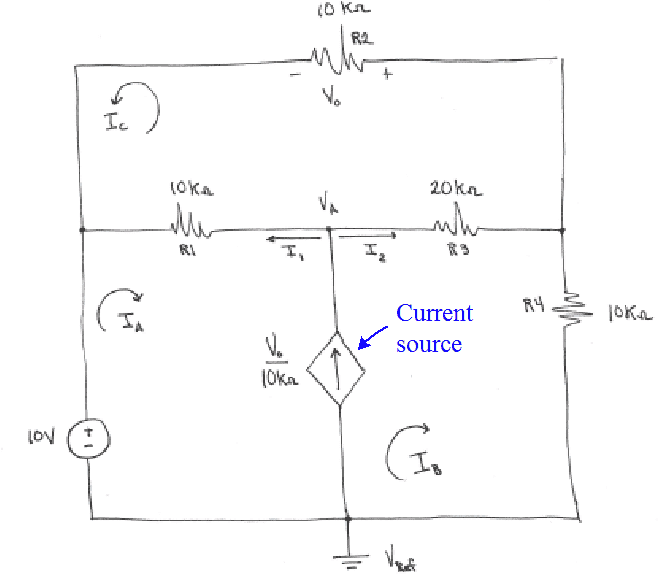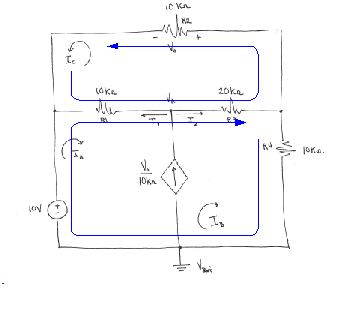# Mesh Analysis

## Homework Statement

Find IA, IB, IC, I1, I2, V0, VA

## The Attempt at a Solution

(V0/10) = IA-IB

For IA: 10v + 10(IA+IC) + (IA-IB)=0
11(IA) - (IB) + 10(IC) = -10v

For IB: -(IB-IA) - 20(IB+IC) - 10(IB) = 0
IA - 31(IB) - 20(IC) = 0

For IC: 10(IA+IC) - 20(IC+IB) - 10(IC) = 0
10(IA) - 20(IB) - 20(IC) = 0

I then put these equations into a matrix (Sorry I don't know how to put them into a matrix on here) and used an online matrix calculator to get the currents:

IA= -0.4A
IB=(18/55)A
IC=(-29/55)A

So then I plugged these values into this formula:
(V0/10) = IA-IB
And got V0= -7.3V

But when I used v=iR to see if I would get the same thing I got

v=(-29/55)(10)= -5.27v

What am I doing wrong?

#### Attachments

• scan0002.pdf
260.5 KB · Views: 145

NascentOxygen
Staff Emeritus
Hi jdawg. Are you able to replace your pdf with a simple jpeg?

gneill
Mentor

## Homework Statement

Find IA, IB, IC, I1, I2, V0, VA

## The Attempt at a Solution

(V0/10) = IA-IB

For IA: 10v + 10(IA+IC) + (IA-IB)=0
11(IA) - (IB) + 10(IC) = -10v
Your term: IA-IB is a current, not a voltage. That won't work for a KVL statement.

The source in the center of the circuit is a current source. It has a value ##V_o / 10kΩ##, which you'll note is a current value. Lying between two meshes as it is, your instinct should be to form a Supermesh to avoid dealing with its unknown potential change in your KVL equations.•jdawg
Oh ok, I retried the problem using super meshes:

First I did a super mesh around the whole circuit:
10+10(IC)-10(IB) = 0
IC = IB - 1 equation1

Then I did a second super mesh around meshes IA and IB excluding IC:
10 - 10(IA+IC) - 20(IC+IB) - 10(IB) = 0
10(IA) + 30(IB) +30(IC) = 10 equation2

And then around IC:
-10(IC) - 10(IC+IA) - 20(IC+IB) = 0
IA +2(IB) +4(IC) = 0 equation3

I then plugged equation 1 into equation 2 and equation 3 and IB ended up canceling out. I'm not sure what I did wrong this time.

gneill
Mentor
Oh ok, I retried the problem using super meshes:

First I did a super mesh around the whole circuit:
10+10(IC)-10(IB) = 0
IC = IB - 1 equation1

Then I did a second super mesh around meshes IA and IB excluding IC:
10 - 10(IA+IC) - 20(IC+IB) - 10(IB) = 0
10(IA) + 30(IB) +30(IC) = 10 equation2

And then around IC:
-10(IC) - 10(IC+IA) - 20(IC+IB) = 0
IA +2(IB) +4(IC) = 0 equation3

I then plugged equation 1 into equation 2 and equation 3 and IB ended up canceling out. I'm not sure what I did wrong this time.
You only need two mesh equations plus the supernode constraint equation. Writing a third mesh equation doesn't provide new information, it just duplicates other information from the other two equations. That's why you found things cancelling out.

So, you previously wrote the required constraint equation relating IA, IB, and IC (via the controlled current supply). Use that in place of one of your mesh equations. Usually the approach is to combine the two loops with the shared current into a supermesh, and write regular mesh equations for any other loops.

Ok so I kept my super mesh 2 and IC equations. I'm still a little confused, so now I just write an equation for either loop IA or IB?

gneill
Mentor
Ok so I kept my super mesh 2 and IC equations. I'm still a little confused, so now I just write an equation for either loop IA or IB?

You can't write equations for loops IA or IB because you don't have a potential for the controlled current source. So you combine IA and IB into a supermesh as you've done, and then write mesh equations for what's left: that's loop IC.•jdawg
So now I have IA+3(IB)+3(IC) = 1 from the super mesh and IA+ 2(IB)+4(IC) = 0 for the IC loop. How do I solve for the three variables with only two equations? Sorry for asking so many questions!

gneill
Mentor
So now I have IA+3(IB)+3(IC) = 1 from the super mesh and IA+ 2(IB)+4(IC) = 0 for the IC loop. How do I solve for the three variables with only two equations? Sorry for asking so many questions!
You've forgotten the supermesh constraint equation.

•jdawg
Thanks so much for all your help!